#### Critical gap events

As the robot moves, several important events can occur in the gap sensor:

1. Disappear: A gap disappears because the robot crosses an inflection ray as shown in Figure 12.28. This means that some previous shadow component is now visible.
2. Appear: A gap appears because the robot crosses an inflection ray in the opposite direction. This means that a new shadow component exists, which represents a freshly hidden portion of the environment.
3. Split: A gap splits into two gaps because the robot crosses a bitangent ray, as shown in Figure 12.29 (this was also shown in Figure 12.5). This means that one shadow component splits into two shadow components.
4. Merge: Two gaps merge into one because the robot crosses a bitangent ray in the oppose direction. In this case, two shadow components merge into one.
This is a complete list of possible events, under a general position assumption that precludes environments that cause degeneracies, such as three gaps that merge into one or the appearance of a gap precisely where two other gaps split.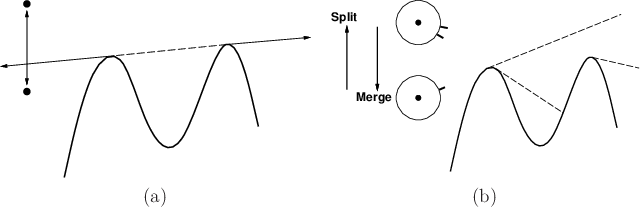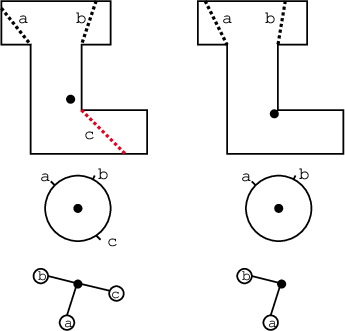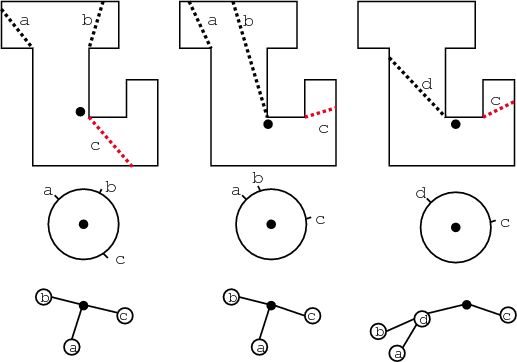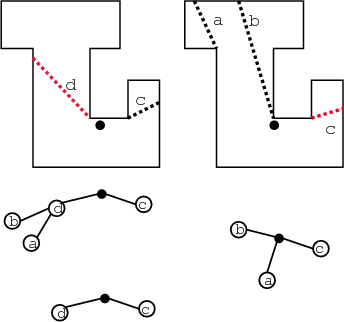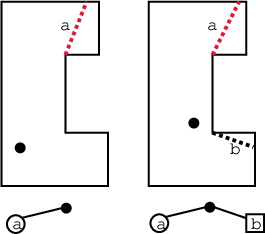As each of these gap events occurs, it needs to be reflected in the tree. If a gap disappears, as shown in Figure 12.30, then the corresponding edge and vertex are simply removed. If a merge event occurs, then an intermediate vertex is inserted as shown in Figure 12.31. This indicates that if that gap is chased, it will split into the two original gaps. If a split occurs, as shown in Figure 12.32, then the intermediate vertex is removed. The appearance of a gap is an important case, which generates a primitive vertex in the tree, as shown in Figure 12.33. Note that a primitive vertex can never split because chasing it will result in its disappearance.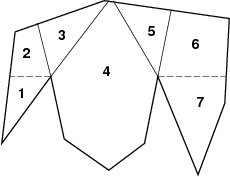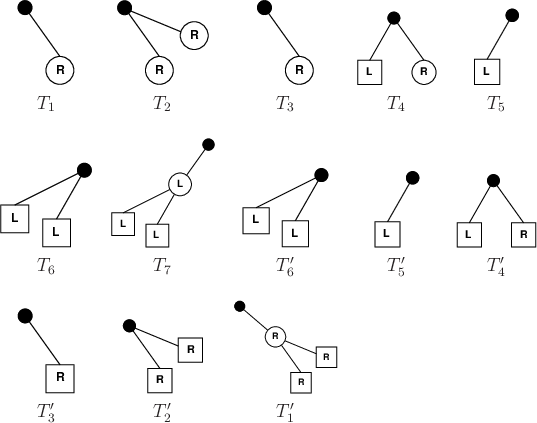A simple example will now be considered.

Example 12..6 (Gap Navigation Tree)   Suppose that the robot does not know the environment in Figure 12.34. It moves from cells 1 to 7 in order and then returns to cell 1. The following sequence of trees occurs: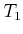,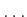,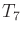,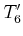,,, as shown in Figure 12.35. The root vertex is shown as a solid black disc. Vertices that are not known to be primitive are shown as circles; primitive vertices are squares. Note that if any leaf vertex is a circle, then it means that the shadow region of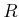that is hidden by that gap has not been completely explored. Note that once the robot reaches cell 5, it has seen the whole environment. This occurs precisely when all leaf vertices are primitive. When the robot returns to the first region, the tree is larger because it knows that the region on the right is composed of two smaller regions to the right. If all leaves are squares, this means that the environment has been completely explored.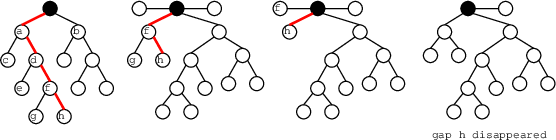In the example, all of the interesting parts of the environment were explored. From this point onward, all leaf vertices will be primitive vertices because all possible splits have been discovered. In a sense, the environment has been completely learned, at the level of resolution possible with the gap sensor. A simple strategy for exploring the environment is to chase any gaps that themselves are nonprimitive leaf vertices or that have children that are nonprimitive leaf vertices. A leaf vertex in the tree can be chased by repeatedly applying actions that chase its corresponding gap in the gap sensor. This may cause the tree to incrementally change; however, there is no problem if the action is selected to chase whichever gap hides the desired leaf vertex, as shown in Figure 12.36. Every nonprimitive leaf vertex will either split or disappear. After all nonprimitive leaf vertices have been chased, all possible splits have been performed and only primitive leaves remain. In this case, the environment has been completely learned.

Steven M LaValle 2020-08-14